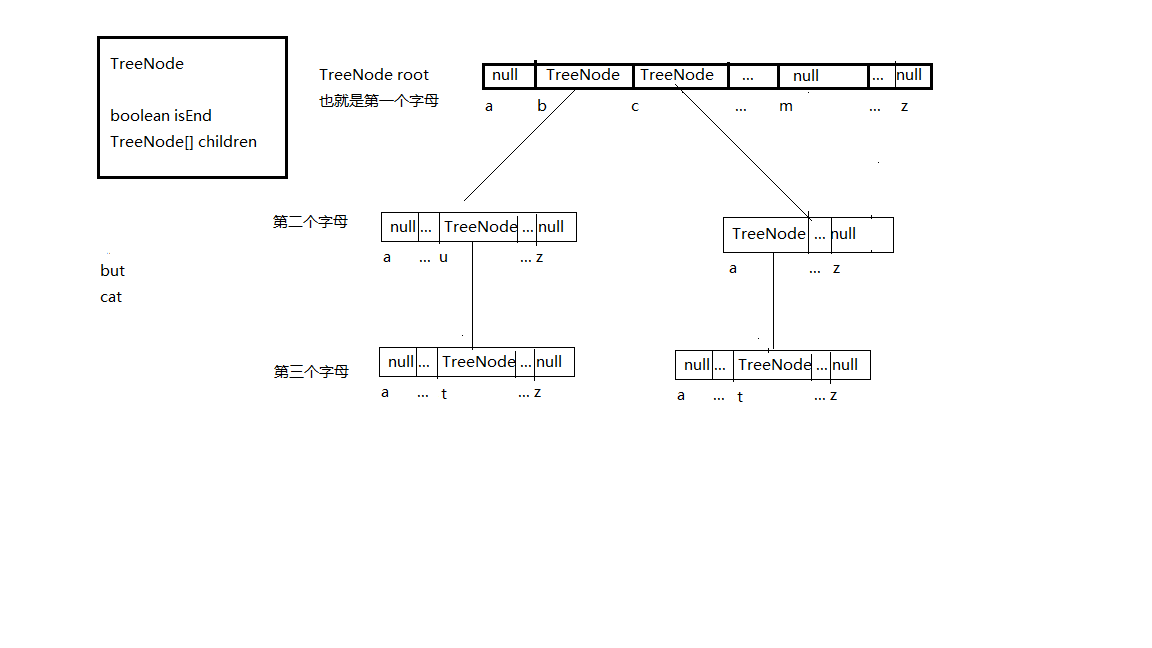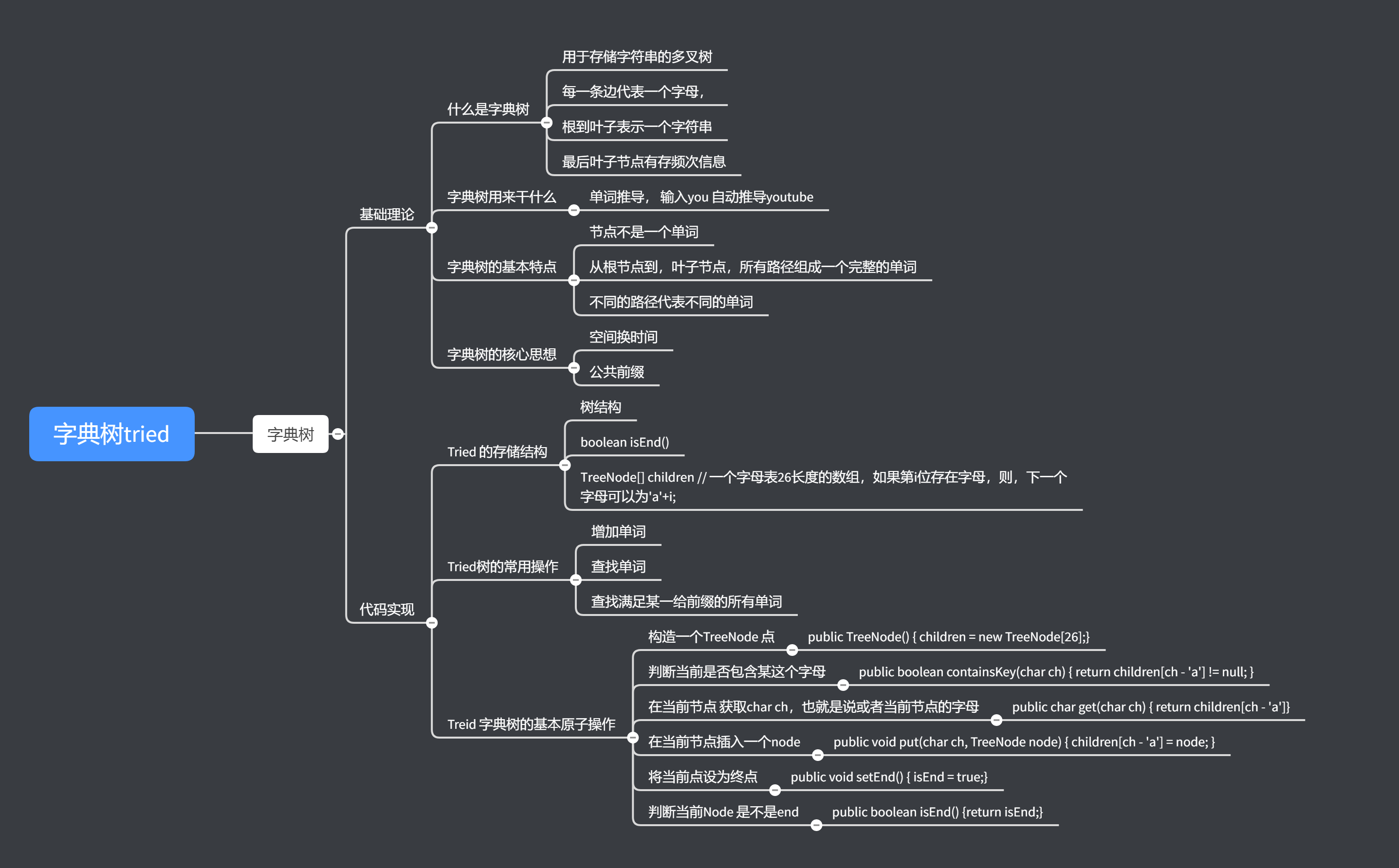Trie 字典树12583基础理论

什么是字典树

1. 用一颗树来存储单词

2. 多叉树每一条边代表一个字母，

3. 根到叶子表示一个字符串

4. 最后叶子节点可以存频次信息

图解﻿

﻿

代码实现

Tried 的存储结构 树结构boolean isEnd()TreeNode[] children // 一个字母表26长度的数组，如果第i位存在字母，则，下一个字母可以为'a'+i;

﻿

Treid 字典树的基本原子操作

public TreeNode() { children = new TreeNode;}

public boolean containsKey(char ch) { return children[ch - 'a'] != null; }在当前节点 获取char ch，也就是说或者当前节点的字母

public char get(char ch) { return children[ch - 'a']}

public void put(char ch, TreeNode node) { children[ch - 'a'] = node; }

public void setEnd() { isEnd = true;}判断当前Node 是不是endpublic boolean isEnd() {return isEnd;}

﻿

Tried树的常用操作

﻿

class Trie {
class TreeNode{
private boolean isEnd = false;
private final int R = 26;
TreeNode[] children;
public TreeNode() {
children = new TreeNode[R];
}
public TreeNode get(char ch) {
return children[ch - 'a'];
}
public void put(char ch, TreeNode node) {
children[ch - 'a'] = node;
}
public void setEnd() {
isEnd = true;
}
public boolean isEnd() {
return isEnd;
}
}
private TreeNode root;
/** Initialize your data structure here. */
public Trie() {
root = new TreeNode();
}
/** Inserts a word into the trie. */
public void insert(String word) {
TreeNode temp = root;
for (int i = 0; i < word.length() ; i++) {
char ch = word.charAt(i);
if (temp.get(ch) == null) {
TreeNode chNode = new TreeNode();
temp.put(ch, chNode);
}
temp = temp.get(ch);
}
temp.setEnd();
}
/** Returns if the word is in the trie. */
public boolean search(String word) {
TreeNode temp = root;
for (int i = 0; i < word.length() ; i++) {
char ch = word.charAt(i);
if (temp.get(ch) == null) {
return false;
}
temp = temp.get(ch);
}
// 那就和下面的寻找前缀没有区别了
if(temp.isEnd() == true) {
return true;
} else {
return false;
}
}
/** Returns if there is any word in the trie that starts with the given prefix. */
public boolean startsWith(String prefix) {
TreeNode temp = root;
for (int i = 0; i < prefix.length() ; i++) {
char ch = prefix.charAt(i);
if (temp.get(ch) == null) {
return false;
}
temp = temp.get(ch);
}
return true;
}
}

思维导图﻿评论